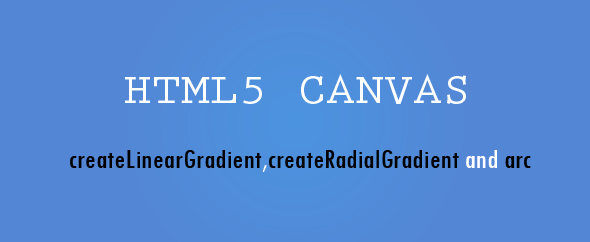Friday, 1 July 2016
In our previous post we have checked some basic shape and functions like line, rect, image, circle etc. Now there is some functions we used without knowing,so we should have a clear idea how about every functions as we will use these function in more advanced way.

In the previous post we created gradient by createLinearGradient and createRadialGradient functions.Both the functions takes 4 and 6 arguments.But you are wondering HOW and WHY?
Let's find out:

Is responsible for creating Linear gradient. It takes 4 arguments like createLinearGradient (0, 0, 500, 300)
The arguments stand for (x0,y0) to (x1,y1);

x0-The x axis of the coordinate of the start point.
y0-The y axis of the coordinate of the start point.
x1-The x axis of the coordinate of the end point.
y1-The y axis of the coordinate of the end point.###### Let’s check with the code:
Assume we have a requirement to have a gradient vertically 300px, so the code will be:
Like the other examples the below two lines are common. var canvas = document.getElementById('canvas');
var getcontext= canvas.getContext('2d');

//Now put the y1 value to the height of the CANVAS, like we have 300 in this scenario.
getcontext.fillStyle = grd;
getcontext.fillRect(0,0,500,300);
###### OUTPUT
See the Pen createLinearGradient by pixel (@pixel-lab) on CodePen.
So, it will come straight 300 vertical gradient.
###### For reverse one the Horizontal:
-- check this example output on the above pen.Just uncomment the above line

###### NOW BOTHWAY
Now I am modifying some color, it will help to understand the further examples.
grd.addColorStop(.5, '#fff'); // new color in the middle
getcontext.fillStyle = grd;
getcontext.fillRect(0,0,500,300);

###### OUTPUT:
Look at the white angle
See the Pen createLinearGradient by pixel (@pixel-lab) on CodePen.

###### Other examples.
Now changing the first two points:
The next one:
###### Check all the example from the above pen. Just uncomment the lines. I belive this will give an advanced idea about the createLinearGradient function.

(x0, y0, r0, x1, y1, r1);
x0-The x axis of the coordinate of the start circle.
y0-The y axis of the coordinate of the start circle.
r0-The radius of the start circle.
x1-The x axis of the coordinate of the end circle.
y1-The y axis of the coordinate of the end circle.
r1-The radius of the end circle.

###### Let’s check with the code:
Now assume we have a requirement that a gradient hold a small circle center of the rectangular.
First I have taken a small 300x300 rectangle and create a small gradient on red over the black screen.
Like the other examples the below two lines are common. var canvas = document.getElementById('canvas');
var getcontext= canvas.getContext('2d');
context.fillStyle = grd;
context.fillRect(0,0,300,300);

###### Explanation:
createRadialGradient(150,150,100,150,150,10);//(x0, y0, r0, x1, y1, r1); Simple putting the gradient 150px on X axis (x0) and 150 Y (y0) axis and its radius 100 (r0) and the inner circle 150 on X axis (x1), 150 Y axis (y1) with inner radius 10 (r1).
###### Another example:
Now with the 500x300 px rectangle.
Initial look with the same code.
Just uncomment the pen
We need to shift the circle to the center, currently we are 500 px of width
So we need to increase the value of x0 and x1 in (x0, y0, r0, x1, y1, r1);So the code now

## Arc

We have used the arc method for creating circle or sphere. It takes 6 arguments like
(x,y,r,start angle,end angle, counterclockwise optional);
x- The x axis of the coordinate of the center of the circle.
y- The y axis of the coordinate of the center of the circle.
start angle - The starting angle
end angle - The ending angle
counterclockwise - Optional. Specifies whether the drawing should be counterclockwise or clockwise. False is default, and indicates clockwise, while true indicates counter-clockwise.
###### Lets play with the code:
I am creating a circle and fill it by a gradient. So the code will be.
var canvas = document.getElementById('canvas');
var getcontext= canvas.getContext('2d');
//same as other functions
getcontext.beginPath();
getcontext.fillStyle= grd;
getcontext.arc(100,75,70,0,2*Math.PI, true);
//(x,y,r,start angle,end angle, counterclockwise optional);
getcontext.fill();
###### OUTPUT
Here x and y simply mentioning the co-ordinates and the r mention the circle radios. You may increase it value based of you requirement. The start angle and end angle you need to mention carefully.
So based if you requirement placed the start and end angle.Now play with the code.
See the Pen Canvas ARC method by pixel (@pixel-lab) on CodePen.
Thanks for visiting.Please support us by shearing our posts and like your social pages.
Please subscribe for our future posts.